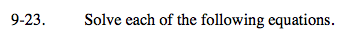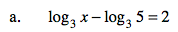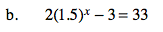### Home > CAAC > Chapter 16 > Lesson 16.9.1.2 > Problem9-23

9-23.$\text{log}_{3} \frac{x}{5}= 2$

$3^2=\frac{x}{5}$